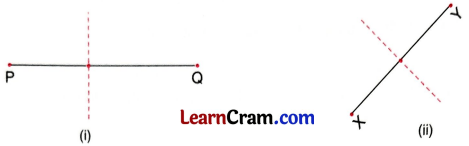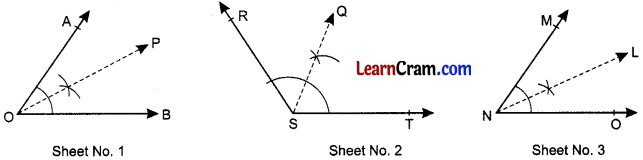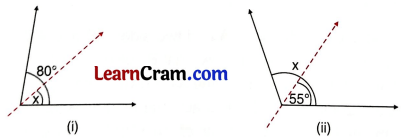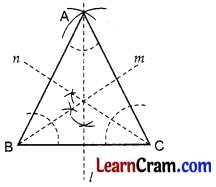# DAV Class 7 Maths Chapter 13 Worksheet 2 Solutions

The DAV Class 7 Maths Book Solutions and DAV Class 7 Maths Chapter 13 Worksheet 2 Solutions of Symmetry offer comprehensive answers to textbook questions.

## DAV Class 7 Maths Ch 13 WS 2 Solutions

Question 1.
Find the line of symmetry of any 3 line segments on different sheets of paper.Draw the perpendicular bisectors of the line segment which is the line of symmetry of the line segment. In above figures;
l1 is the line of symmetry of $$\overline{\mathrm{AB}}$$
l2 is the line of symmetry of $$\overline{\mathrm{PQ}}$$
and l3 is the line of symmetry of $$\overline{\mathrm{MN}}$$.

Question 2.
Check if the dotted lines are the line of symmetry of the given line segments.(i) The dotted line is not the perpendicular bisector of PQ, so it is not a line of symmetry.
(ii) The dotted line is the perpendicular bisector of XY, so it is a line of symmetry.Question 3.
Lines l and m are the lines of symmetry of the line segments XY and YZ respectively. If XA = 4 cm and YZ = 6 cm, find AY, YB, XZ.l is the line of symmetry of XY.
∴ XA = AY
⇒ 4 = AY
⇒ AY = 4 cm

m is the line of symmetry of YZ, YZ = 6 cm
∴ YB = BZ = $$\frac{6}{2}$$ = 3 cm
XZ = 2XA + YZ = 2(4) + 6 = 8 + 6 = 14 cm
Hence, AY = 4 cm, YB = 3 cm and XZ = 14 cm

Question 4.
Find the line of symmetry of the following angles on different sheets of paper.
(a) 60°
(b) 150°
(c) 45°(a) OP is the bisector of ∠AOB, so it is the line of symmetry of ∠AOB where ∠AOB = 60°
(b) SQ is the bisector of ∠RST, so it is the line of symmetry of ∠RST, where ∠RST = 150°
(c) NL is the bisector of ∠MNO, so it is the line of symmetry of ∠MNO, where ∠MNO = 45°.

Question 5.
If dotted lines represent the lines of symmetry of the given angles, find x in each case.In fig. (z) The dotted line is the bisector of the angle
x = $$\frac{80^{\circ}}{2}$$ = 40°
In figure (ii) the dotted line is the bisector of the angle
x = 2 × 55° = 110°

Question 6.
The dotted line represents the line of symmetry of ΔABC. If ∠ABC = 40° and OB = 4.5 cm, find.(a) ∠BAO
(b) Measure of OC. Give reasons.
(a) In ΔABC, OA is the line of symmetry 8
∴ ΔABC is an isosceles triangle ∠B = ∠C
∠A + ∠B + ∠C = 180°
∠A + 40° + 40° = 180°
∴ ∠A = 180° – 80° = 100°
AO is the line of symmetry
AO is the bisector of ∠A
∴ ∠BAO = $$\frac{100^{\circ}}{2}$$ = 50°

(b) OA is the line of symmetry of BC
OA is the perpendicular bisector of BC BO = OC = 4.5 cm
Hence, ∠BAO = 50° and OC = 4.5 cmQuestion 7.
Construct an equilateral ΔABC of side 4 cm. Find all its line of symmetry.Draw ΔABC in which AB = BC = AC = 4 cm.
Draw the bisectors of ∠A, ∠B and ∠C
Al, Bm and Cn are the line of symmetry of an equilateral ΔABC.

Question 8.
Will a scalene triangle have any line of symmetry?